• 链表是一种数据结构，和数组同级。接下来通过本文给大家介绍Java单链表基本操作的实现，非常不错，具有参考借鉴价值，感兴趣的朋友一起看下吧
•线性链表
• 实验一 单链表 #include "stdio.h" #include "stdlib.h" typedef int ElemType; typedef struct LNode { ElemType data; struct LNode *next; }LNode*LinkList; void creatLNode(LinkList &head) { int i,n; LNode *...
• 单链表基本操作 1.头插法建立单链表 2.尾插法建立单链表 3.查找结点 3.修改结点 4.插入结点 5.删除结点 本篇只有c语言代码，具体思路讲解请看这篇博客：数据结构-线性结构-单链表 1.头插法建立单链表 #include<...
单链表基本操作
1.头插法建立单链表 2.尾插法建立单链表 3.查找结点 3.修改结点 4.插入结点 5.删除结点
本篇只有c语言代码，具体思路讲解请看这篇博客：数据结构-线性结构-单链表
1.头插法建立单链表
#include<stdio.h>
#include<stdlib.h>

//单链表的结构定义
typedef struct LNode
{
int data;			//data存放结点数据域
struct LNode *next;	//指向后继结点的指针
}LNode;					//定义单链表结点类型

//头插法建立单链表
void createlistF(LNode *&L, int a[], int n)
{
LNode *s;
int i;
L = (LNode*)malloc(sizeof(LNode));
L ->next = NULL;
for(i=0;i<n;++i)
{
s = (LNode*)malloc(sizeof(LNode));
s ->data = a[i];
//下面两句为头插法的关键步骤
s ->next = L ->next;	//s所指新结点的指针域next指向L中的开始结点
L ->next = s;			//头结点的指针域next指向s结点，使得s成为新的开始结点

}

}

//打印链表数据
void printfList(LNode *L)
{
LNode *temp = L;
int count = 0;
printf("表中的元素为:\n");
while(temp->next)
{
temp = temp->next;
printf("%d\t",temp->data);
count++;
if(count%5==0)
{
printf("\n");
}
}
printf("\n");

}

int main()
{
LNode *L;
int n;
printf("请输入数组的个数：");
scanf("%d",&n);
int a[n];
printf("请输入数组中的数(用空格分开)：\n");
for(int i=0;i<n;++i)
{
scanf("%d",&a[i]);
}

//测试头插法建立链表
createlistF(L,a,n);

//查看建立后的链表
printfList(L);

return 0;
}


运行结果如下：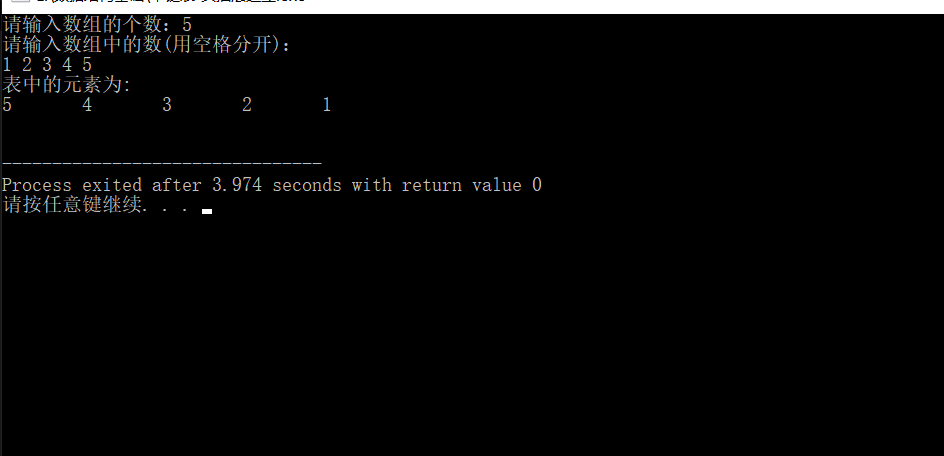2.尾插法建立单链表
#include<stdio.h>
#include<stdlib.h>

typedef struct LNode
{
int data;			//data中存放结点的数据域
struct LNode *next;	//指向后继结点的指针
}LNode; 				//定义单链表结点类型

//尾插法建立链表
void createlistR(LNode *&L, int a[], int n) //要改变的变量用引用型
{
LNode *s,*r;		//s用来指向新申请的结点，r之中指向L的终端结点
int i;
L = (LNode *)malloc(sizeof(LNode));		//申请L的头结点空间
L -> next = NULL;
r = L;				// r指向头结点，因为此时头结点就是终端结点
for(i=0;i<n;++i)	// 循环申请n个结点，来接受数组a中的元素
{
s = (LNode*)malloc(sizeof(LNode));	// s指新申请的结点
s ->data = a[i];// 用新申请的结点来接受a中的一个元素
r ->next = s;	// 用来接纳新结点
r = r ->next;	// r指向终端结点，以便于接纳下一个到来的结点
}
r ->next = NULL;	// 数组a中所有的元素都已经装入链表L中，L的终端结点的指针域置为NULL，L建立完成
}

//打印链表数据
void printfList(LNode *L)
{
LNode *temp = L;
int count = 0;		//计数器
printf("表中的元素为:\n");
while(temp->next)
{
temp = temp->next;
printf("%d\t",temp->data);
count++;
if(count%5==0)		//每5个元素作为一行
{
printf("\n");
}
}
printf("\n");

}

int main()
{
LNode *L;
int n;
printf("请输入数组的个数：");
scanf("%d",&n);
int a[n];
printf("请输入数组中的数(用空格分开)：\n");
for(int i=0;i<n;++i)
{
scanf("%d",&a[i]);
}

//测试尾插法建立链表
createlistR(L,a,n);

//查看建立后的链表
printfList(L);

return 0;

}

运行结果如下：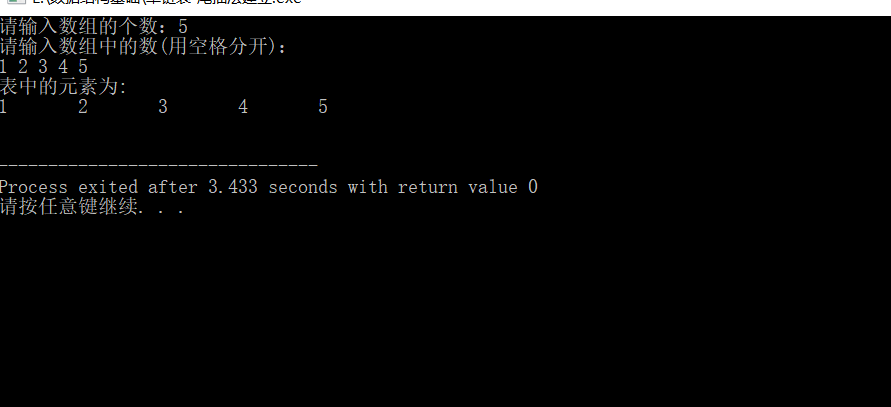3.查找结点
#include<stdio.h>
#include<stdlib.h>

//单链表的结构定义
typedef struct LNode
{
int data;			//data存放结点数据域
struct LNode *next;	//指向后继结点的指针
}LNode;					//定义单链表结点类型

//头插法建立单链表
void createlistF(LNode *&L, int a[], int n)
{
LNode *s;
int i;
L = (LNode*)malloc(sizeof(LNode));
L ->next = NULL;
for(i=0;i<n;++i)
{
s = (LNode*)malloc(sizeof(LNode));
s ->data = a[i];
s ->next = L ->next;
L ->next = s;

}

}

//打印链表数据
void printfList(LNode *L)
{
LNode *temp = L;
int count = 0;		//计数器
printf("表中的元素为:\n");
while(temp->next)
{
temp = temp->next;
printf("%d\t",temp->data);
count++;
if(count%5==0)		//每5个元素作为一行
{
printf("\n");
}
}
printf("\n");

}

//在链表L中查找与e相等的元素，找到的话返回其位置， 否则返回未找到。
int searchElem(LNode *L, int e)
{
LNode *temp = L;
int i = 1;
int p = 0;
while(temp ->next)
{
temp = temp ->next;
if(e == temp->data)
{
p = i;
printf("找到了与%d相等元素位置为%d\n",e,p);
return 1;
}
i++;
}
printf("抱歉，没有找到！");
return -1;
}

int main()
{
LNode *L;
int n;
printf("请输入数组的个数：");
scanf("%d",&n);
int a[n];
printf("请输入数组中的数(用空格分开)：\n");
for(int i=0;i<n;++i)
{
scanf("%d",&a[i]);
}
createlistF(L,a,n);
printfList(L);

//测试查找结点
int e;
printf("请输入要查找的结点:");
scanf("%d",&e);
searchElem(L,e);

return 0;
}


运行结果如下：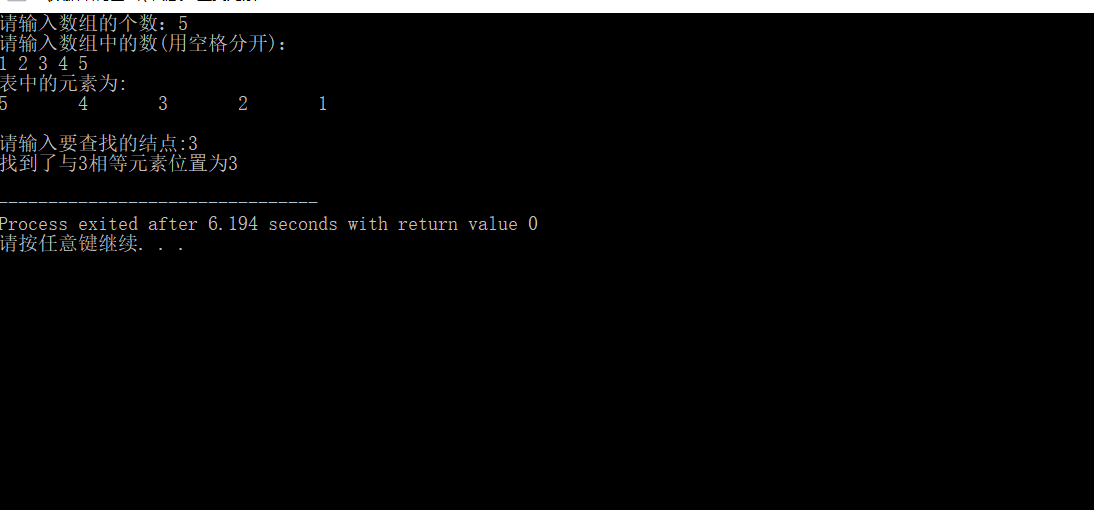4.修改结点
#include<stdio.h>
#include<stdlib.h>

//单链表的结构定义
typedef struct LNode
{
int data;			//data存放结点数据域
struct LNode *next;	//指向后继结点的指针
}LNode;					//定义单链表结点类型

//头插法建立单链表
void createlistF(LNode *&L, int a[], int n)
{
LNode *s;
int i;
L = (LNode*)malloc(sizeof(LNode));
L ->next = NULL;
for(i=0;i<n;++i)
{
s = (LNode*)malloc(sizeof(LNode));
s ->data = a[i];
s ->next = L ->next;
L ->next = s;

}

}

//打印链表数据
void printfList(LNode *L)
{
LNode *temp = L;
int count = 0;		//计数器
printf("表中的元素为:\n");
while(temp->next)
{
temp = temp->next;
printf("%d\t",temp->data);
count++;
if(count%5==0)		//每5个元素作为一行
{
printf("\n");
}
}
printf("\n");

}

// 修改单链表L的第p个位置上的结点
int replace(LNode *L, int p, int e)
{
LNode *temp = L;
temp = temp ->next;
for(int i=1;i<p;++i)
{
temp = temp ->next;
}
temp ->data = e;
}

int main()
{
LNode *L;
int n;
printf("请输入数组的个数：");
scanf("%d",&n);
int a[n];
printf("请输入数组中的数(用空格分开)：\n");
for(int i=0;i<n;++i)
{
scanf("%d",&a[i]);
}
createlistF(L,a,n);
printfList(L);

//测试修改结点
int p,e;
printf("请输入要修改的位置和更改后的元素(用空格分开)：\n");
scanf("%d %d",&p,&e);
replace(L,p,e);

//修改后打印链表数据
printfList(L);

return 0;
}


运行结果如下：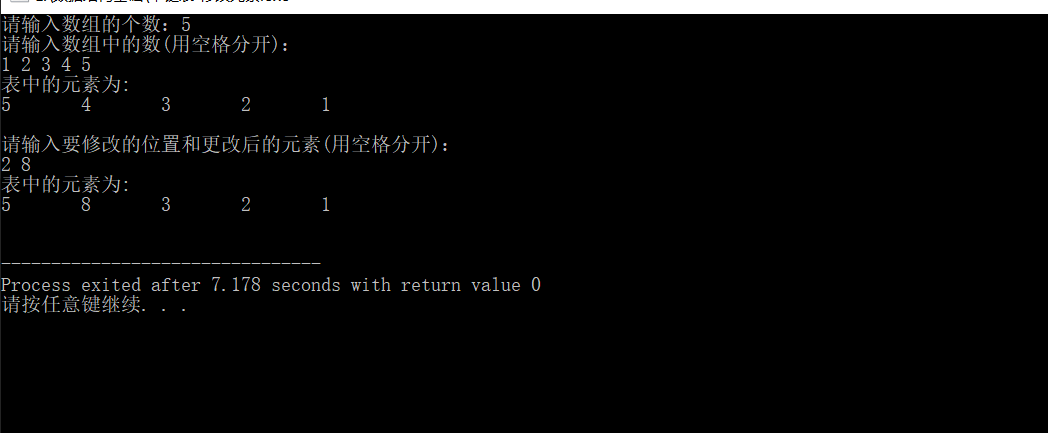5.插入结点
#include<stdio.h>
#include<stdlib.h>

//单链表的结构定义
typedef struct LNode
{
int data;			//data存放结点数据域
struct LNode *next;	//指向后继结点的指针
}LNode;					//定义单链表结点类型

//头插法建立单链表
void createlistF(LNode *&L, int a[], int n)
{
LNode *s;
int i;
L = (LNode*)malloc(sizeof(LNode));
L ->next = NULL;
for(i=0;i<n;++i)
{
s = (LNode*)malloc(sizeof(LNode));
s ->data = a[i];
s ->next = L ->next;
L ->next = s;

}

}

//打印链表数据
void printfList(LNode *L)
{
LNode *temp = L;
int count = 0;		//计数器
printf("表中的元素为:\n");
while(temp->next)
{
temp = temp->next;
printf("%d\t",temp->data);
count++;
if(count%5==0)		//每5个元素作为一行
{
printf("\n");
}
}
printf("\n");

}

//在链表L的第p个位置上插入元素e
void insertElem(LNode *L, int p, int e)
{
LNode *temp = L;
int i = 0;
while(i<p-1)
{
temp = temp ->next;
++i;
}
LNode *s = (LNode*)malloc(sizeof(LNode)); //创建新结点
s ->data = e;
s->next = temp ->next;
temp ->next = s;
}

int main()
{
LNode *L;
int n;
printf("请输入数组的个数：");
scanf("%d",&n);
int a[n];
printf("请输入数组中的数(用空格分开)：\n");
for(int i=0;i<n;++i)
{
scanf("%d",&a[i]);
}
createlistF(L,a,n);
printfList(L);

//测试插入结点
int p,e;
printf("请输入要插入的位置和要插入的元素(用空格分开)：\n");
scanf("%d %d",&p,&e);
insertElem(L,p,e);

//插入后打印链表数据
printfList(L);

return 0;
}


运行结果如下：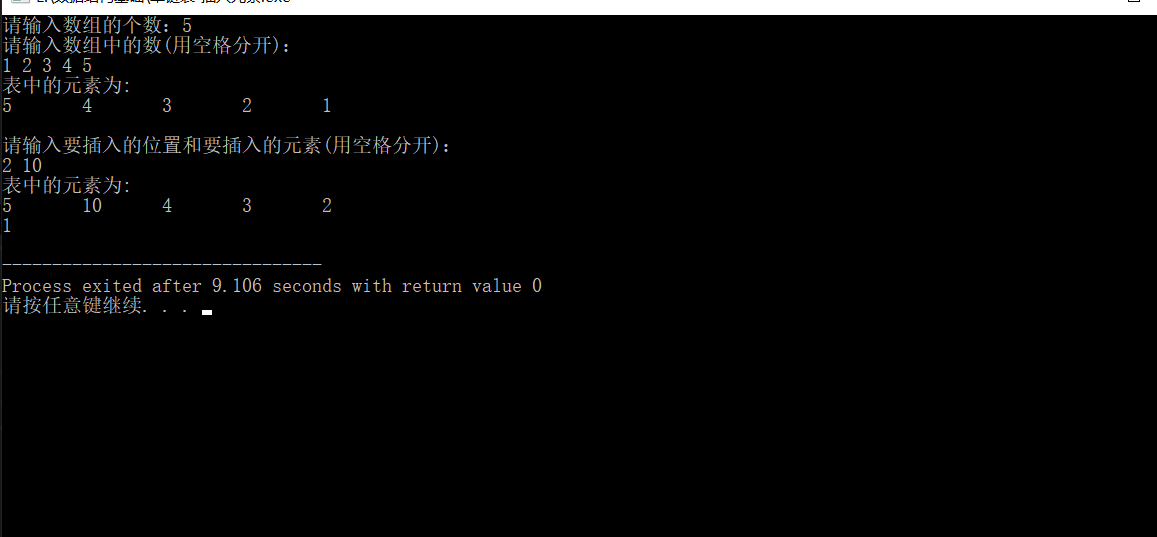6.删除结点
#include<stdio.h>
#include<stdlib.h>

//单链表的结构定义
typedef struct LNode
{
int data;			//data存放结点数据域
struct LNode *next;	//指向后继结点的指针
}LNode;					//定义单链表结点类型

//头插法建立单链表
void createlistF(LNode *&L, int a[], int n)
{
LNode *s;
int i;
L = (LNode*)malloc(sizeof(LNode));
L ->next = NULL;
for(i=0;i<n;++i)
{
s = (LNode*)malloc(sizeof(LNode));
s ->data = a[i];
s ->next = L ->next;
L ->next = s;

}

}

//打印链表数据
void printfList(LNode *L)
{
LNode *temp = L;
int count = 0;		//计数器
printf("表中的元素为:\n");
while(temp->next)
{
temp = temp->next;
printf("%d\t",temp->data);
count++;
if(count%5==0)		//每5个元素作为一行
{
printf("\n");
}
}
printf("\n");

}

//删除单链表L中第p位置上的结点
void deleteElem(LNode *L, int p, int *e)
{
LNode *temp = L;
int i = 0;
//找到删除结点的上一结点，即第p-1个结点
while(i<p-1)
{
temp = temp ->next;
++i;
}
LNode *q = temp->next;	//定义一个q指针指向被删除结点
*e = q->data;			//保存被删除的结点的数据域
temp->next = q->next;	//删除结点的上一个结点的指针域指向删除结点的下一个结点
free(q);				//释放q所指结点的内存空间
}

int main()
{
LNode *L;
int n;
printf("请输入数组的个数：");
scanf("%d",&n);
int a[n];
printf("请输入数组中的数(用空格分开)：\n");
for(int i=0;i<n;++i)
{
scanf("%d",&a[i]);
}
createlistF(L,a,n);
printfList(L);

//测试删除结点
int p,e;
e = NULL;
printf("请输入要删除结点的位置：\n");
scanf("%d",&p);
deleteElem(L,p,&e);

//插入后打印链表数据
printfList(L);

return 0;
}


运行结果如下：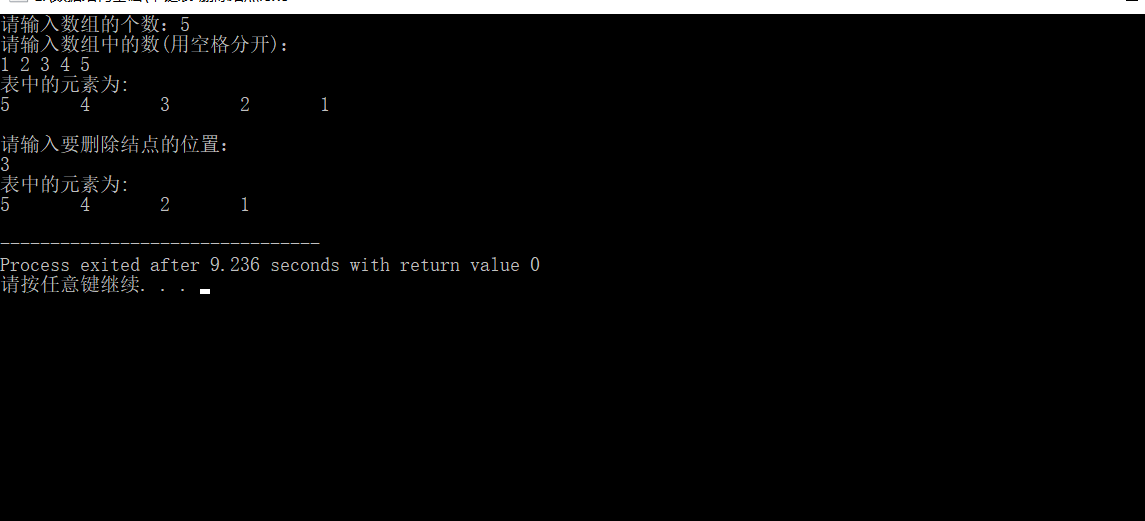展开全文c语言
• 1.单链表基本操作 单链表节点结构 package demo16; //单链表节点结构 //java中单链表节点使用Node实体类表示，只有后续没有前继 public class Node { Node next=null;//next指针指向下一个节点，初始为null int...
1.单链表基本操作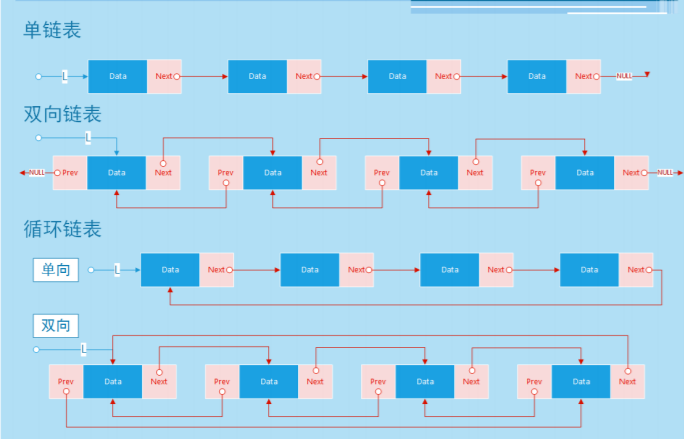链表的核心操作集有 3 种：插入、删除、查找(遍历）单链表 [Linked List]：由各个内存结构通过一个 Next 指针链接在一起组成，每一个内 存结构都存在后继内存结构(链尾除外），内存结构由数据域和 Next 指针域组成。
单链表实现图示：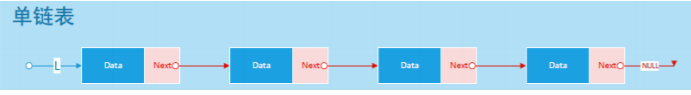解析：
Data 数据 + Next 指针，组成一个单链表的内存结构 ； 第一个内存结构称为 链头，最后一个内存结构称为 链尾； 链尾的 Next 指针设置为 NULL [指向空]； 单链表的遍历方向单一（只能从链头一直遍历到链尾）
单链表节点结构
package demo16;
//单链表节点结构
//java中单链表节点使用Node实体类表示，只有后续没有前继
public class Node {
Node next=null;//next指针指向下一个节点，初始为null
int data; //节点数据

public Node() { }

public Node( int data) {
this.data = data;
}
}

单链表基本操作
package demo16;
//单链表的增删改查

//头指针:标识头结点(始终指向)，单链表每次操作都要从头结点开始，初始化为null

/**
* 添加节点
* 创建一个节点，判断是否有头节点，没有头节点那么创建的节点就是头节点，
* 下一次再创建节点，头节点已经有了，就从头节点循环遍历直到最后一个节点，
* 然后把新创建的节点挂在最后一个节点上。
*
* @param data
*/
Node node = new Node(data);//创建节点，向节点存入数据
//节点接入链表中
//若是空链表
return;
}
//若不是空链表
while (temp.next != null) {
temp = temp.next; //向后移动一个节点,temp指向当前节点的下一个节点
}
//找到链表最后一个节点，将新节点挂在其后
temp.next = node;
}

/**
* 删除节点
* 1.判断删除的节点是否在链表中
* 2.判断删除的链表是否是头结点，是则更新头结点，不是则遍历，通过index确定删除节点
* 3.由于不是双链表，需要变量时刻记录当前节点curNode的前一个节点preNode
*
* @param index
* @return
*/
public boolean deleteNode(int index) {
if (index < 1 || index > nodeLength()) return false; //节点不存在
if (index == 1) {//删除头结点
return true;
}
int count = 2;//标识当前节点在链表中位置
while (curNode != null) {
if (count == index) {//找到节点
preNode.next = curNode.next;//preNode指向当前节点下一个节点完成删除
return true;
}
//当前节点不是目标节点，则preNode和curNode分别后移分别指向各自下一个节点
preNode = preNode.next;
curNode = curNode.next;
count++;
}
return true;
}

/**
* 链表长度
*
* @return
*/
public int nodeLength() {
int count = 1;//计数器
if (head == null) return 0;//空链表
while (temp.next != null) {
temp = temp.next;//temp指向下一个节点
count++;
}
return count;
}

/**
* 输出链表
* 1.先判断链表是否为空，不为空则遍历链表
*/
while(temp!=null){
System.out.print(temp.data+" ");
temp=temp.next;
}
System.out.println();
}
}
测试类
package demo16;

public class Test {
public static void main(String[] args) {
//添加链表节点
}
}


展开全文数据结构 java
• ## C语言单链表基本操作总结

万次阅读 多人点赞 2018-03-26 00:08:23
C语言单链表基本操作 本文是参考他人实现的C语言单链表，对多篇博文整理的结果，仅作为学习笔记。文末有参考出处。1、单链表定义 链表是通过一组任意的存储单元来存储线性表中的数据元素，这些存储单元可以是连续...

C语言单链表基本操作

本文是参考他人实现的C语言单链表，对多篇博文整理的结果，仅作为学习笔记。文末有参考出处。

1、单链表定义

链表是通过一组任意的存储单元来存储线性表中的数据元素，这些存储单元可以是连续的也可以是不连续的。为了建立起数据元素之间的关系，对于每个数据元素除了存放数据元素自身的信息外，还必须有包含的指示该元素直接后继元素存储位置的信息，这两部分信息组成一个结点，即每个结点都有至少包括两个域，一个域存储数据元素信息，称为
数据域，另一个域存储直接后继的地址,称为
指针域。

typedef struct node{
int data;    //数据域
struct node *next;    //指针域
}NODE;

2、函数声明

typedef struct node{
int data;
struct node *next;
}Node, *pNode;

//初始化一个链表结点
pNode init_node(Node *pnode, int data);
//创建链表函数
pNode create_list();
//遍历链表
//头插法建立链表，每次在表头插入
//尾插法建立链表
//求链表长度
//按值查找操作
//前插法
pNode insertnode_bypre(Node *phead, Node node, int data, int key);
//后插法
pNode insertnode_byback(Node *phead, Node node, int data, int key);
//删除结点
//链表倒置
//链表排序（冒泡排序）
pNode linksort(Node *phead);
3、具体实现

初始化一个链表结点

//初始化一个链表结点
pNode init_node(Node *pnode, int data)
{
pnode = (Node *)malloc(sizeof(Node));
pnode->data = data;//初始化数字域
pnode->next = NULL;//初始化指针域

return pnode;
}


//创建链表函数
pNode create_list();

//创建链表函数
pNode create_list()
{
int i;                                            //    用于下面循环
int len;                                        //    用来存放有效节点的字数
int val;                                        //    用于临时存放用户输入的数据
pNode pHead = (pNode)malloc(sizeof(Node));        //  分配一个不存放有效数据的头结点
pNode pTail = pHead;                            //    链表的最后一个节点
pTail->next = NULL;                            //    最后一个节点的指针置为空
printf("请输入节点个数：");
scanf("%d", &len);
for (i = 0; i < len; i++)
{
printf("第 %d 个节点的数值：", i + 1);
scanf("%d", &val);
pNode pNew = (pNode)malloc(sizeof(Node));    //    为节点分配空间
pNew->data = val;                            //将用户输入的数据赋给节点的成员
pTail->next = pNew;                        //将最后一个节点的指针指向下一个新的节点
pNew->next = NULL;                            //将新节点中的指针置为空
pTail = pNew;                                //将新节点赋给最后的一个节点
}

}

//遍历链表

//遍历链表
{
while (NULL != p)                                //节点p不为空，循环
{
printf("%d ", p->data);
p = p->next;
}
printf("\n");
return;
}



//头插法建立链表，每次在表头插入

//头插法建立链表，每次在表头插入
{
Node *pnode = init_node(&node, data);

{
return pnode;
}
else
{
pnode->next = ptmp;
}

}

//尾插法建立链表

//尾插法建立链表
{
Node *pnode = init_node(&node, data);

{
return pnode;
}
else
{
while (ptmp->next != NULL)
{
ptmp = ptmp->next;
}
ptmp->next = pnode;
}

}

//求链表长度

//求链表长度
{
int len = 0;

while (ptmp != NULL)
{
len++;
ptmp = ptmp->next;
}

return len;
}


//按值查找操作

//按值查找操作
{

if (ptmp == NULL)
{
return NULL;
}

while (ptmp->data != key && ptmp->next != NULL)
{
ptmp = ptmp->next;
}

if (ptmp->data == key)
{
return ptmp;
}
if (ptmp->next == NULL)
{
return NULL;
}
}

//前插法
pNode insertnode_bypre(Node *phead, Node node, int data, int key);

//前插法
pNode insertnode_bypre(Node *phead, Node node,int data,int key)
{
Node *pnode = init_node(&node, data);//初始化插入的结点

{
return pnode;
}
{
pnode->next = ptmp;
}
else
{
while((ptmp->next != NULL) && (ptmp->next->data != key))
{
ptmp = ptmp->next;
}
if (ptmp->next == NULL)//没有找到的情况
{
}
else//把新结点插入到目标结点的前面
{
ptmp->data = key;
pnode->next = ptmp->next;
ptmp->next = pnode;
}
}

}


//后插法
pNode insertnode_byback(Node *phead, Node node, int data, int key);

//后插法
pNode insertnode_byback(Node *phead,Node node ,int data,int key)
{
Node *pnode = init_node(&node, data);//初始化插入的结点

if (ptmp == NULL)//链表为空，或者没有找到
{
}
if (ptmp->next == NULL)//如果key为最后一个结点
{
ptmp->next = pnode;
}
else//将新结点插入到目标结点的后面
{
pnode->next = ptmp->next;
ptmp->next = pnode;
}

}



//删除结点

//删除结点
{
Node *tmp = NULL;

{
return NULL;
}
{
free(ptmp);
ptmp = NULL;
}
else
{
while (ptmp->next != NULL && ptmp->next->data != key)//没找&&没有找到
{
ptmp = ptmp->next;
}
if (ptmp->next == NULL)//没有找到
{
}
if (ptmp->next->data == key)//找到目标结点删除之
{
tmp = ptmp->next;

ptmp->next = tmp->next;
free(tmp);
tmp = NULL;
}
}

}


//链表倒置

//链表倒置
//链表的倒置算法思路：一次取出原链表中的每一个结点，将
//其作为第一个结点插入新链表中。
{
Node *ptmp = NULL;//创建一个新链表
Node *tmp = NULL;

{
printf("list is empty!\n");
return NULL;
}
else
{
{
tmp->next = ptmp;
ptmp = tmp;
}
}

}



//链表排序（冒泡排序）

//链表排序（冒泡排序）
{
Node *p = NULL;//i
Node *q = NULL;//j
Node *r = NULL;
Node tmp;

for (p = phead; p; p = p->next)
{
for (q = p->next; q; q = q->next)
{
if (p->data > q->data)
{
tmp = *p;
*p = *q;
*q = tmp;

r = p->next;
p->next = q->next;
q->next = r;
}
}
}

}

4、测试代码

主函数

//主函数
int main(int argc, int argv[])
{
int opt = 0;

printf("请创建一个链表：\n");

printf("遍历链表：\n");
/*......TO DO......*/
return 0;
}



参考链接：

1、https://blog.csdn.net/men_wen/article/details/52877331?locationNum=14&fps=1

2、https://www.cnblogs.com/chenxiaohei/p/6862791.html


展开全文C语言
• 实验二 单链表基本操作的实现 一、实验学时： 2学时 二、实验目的 实现单链表的基本操作 三、实验内容 单链表的建立、取指定元素、返回指定元素位置 单链表中插入新元素、删除指定元素操作的实现 四、主要仪器...
实验二 单链表基本操作的实现
一、实验学时： 2学时
二、实验目的
实现单链表的基本操作
三、实验内容
单链表的建立、取指定元素、返回指定元素位置单链表中插入新元素、删除指定元素操作的实现
四、主要仪器设备及耗材
硬件：计算机一台软件：VC++ 6.0，MSDN2003或者以上版本
五、实验步骤
分析问题写出算法编制程序上机调试分析结果
六、程序清单
#include<stdio.h>
#include<string.h>
#include<stdlib.h>
#define OK 0
#define ERROR -1
typedef int Status;

//用户自定义类型Book
typedef struct
{
char no;	//图书编号
char name;	//书名
float price;	//价格
}Book;

//创建单链表
typedef struct LNode
{
Book data;		//存Book类型的数据
struct LNode *next;	//指向下一个Book

//单链表初始化
{
//构造一个空的单链表L
L=new LNode;	//生成新结点作为头结点，用头指针L指向头结点
L->next=NULL;	//头结点的指针域置空
return OK;
}

//取值
{
//在带头结点的单链表L中根据序号i获取元素的值，用e返回L中第i个数据元素的值
LNode *p;
p=L->next;	//初始化，p指向首元结点，计数器j初值赋为1
int j=1;
while(p&&j<i)	//顺序链向后扫描，直到p为空或p指向第i个元素
{
p=p->next;	//p指向下一个节点
++j;		//计数器j相应加1
}
if(!p||j>i) return ERROR;	//i值不合法i>n 或 i≤0
e=p->data;	//取第i个结点的数据域
return OK;
}

//查找
{
在带头结点的单链表L中查找值为e的元素
LNode *p;
p=L->next;	 //初始化，p指向首元结点
while(p&&(strcmp(p->data.no,e.no)!=0||strcmp(p->data.name,e.name)!=0||p->data.price!=e.price))	//顺序链向后扫描，直到p为空或p所指结点的数据域的内容等于e
p=p->next;	//p指向下一个结点
return p;	 //查找成功返回值为e的结点地址p，查找失败p为NULL
}

//插入
{
//在带头结点的单链表L中第i个位置插入值为e的新结点
LNode *p,*s;
p=L;int j=0;
while(p&&(j<i-1))
{p=p->next;j++;}	//查找第i个结点，p指向该结点
if(!p||j>i-1) return ERROR;	//i>n+1或者i<1
s=new LNode;	//生成新结点*s
s->data=e;	 //将结点*s的数据域置为e
s->next=p->next;	//将结点*s的指针域指向p的下一个结点
p->next=s;	//将结点*p的指针域指向*s
return OK;
}

//删除
{
LNode *p,*q;
p=L;int j=0;
while((p->next) && (j<i-1))	//查找第i-1个结点，p指向该结点
{p=p->next;++j;}
if(!(p->next)||(j>i-1)) return ERROR;	//超出范围
q=p->next;	//临时保存一下被删结点
p->next=q->next;	//p的后继指向q的后继(p的后继的后继)
delete q;	//释放空间
}

int main()
{
//调用初始化
InitList(L);
Book b1={"10101","计算机网络",32.5};
Book b2={"10102","计算机组成原理",46.8};
//调用插入
ListInsert(L,1,b1);
ListInsert(L,2,b2);
//调用取值
Book b;
GetElem(L,1,b);
printf("%s,%s,%-5.2f\n",b.no,b.name,b.price);
//调用查找
LNode *p1=LocateElem(L,b1);
printf("查到的第一本书为：\n%s,%s,%-5.2f\n",p1->data.no,p1->data.name,p1->data.price);

//调用删除
//ListDelete(L,1);

printf("\n----------------------------\n");
while(L=L->next)
{
printf("%s,%s,%-5.2f\n",L->data.no,L->data.name,L->data.price);
}
}

七、运行结果及分析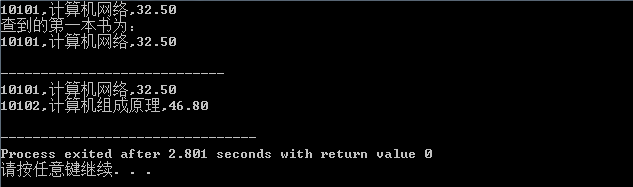八、小总结
这个在建立的时候就和顺序表有所不同 顺序表是里面的elem指向数组，还有个成员就是length，就和数组用着差不多；而链表里的data里存的是Book结构体，还有个指针next，它指向下一个结构体，链表嘛，拿个链子拴起来了。初始化：开一个Book结构体的空间，然后用指针指它，也就是头指针指向头结点。然后把头结点的指针域置空。 初始化就完成了。插入：把传过来的L赋给p，然后通过while循环找到位置i-1，之后开一个空间给s，把s的数据域置为元素e，（p是i-1的位置，s要到i的位置）s的后继指向p的后继，p的后继指向s。删除：把传过来的L赋给p，然后通过循环找到位置i，注意这里是++j，和插入时的j++不同，那是找i-1位置，这是找i位置，之后q指向p的后继，p的后继指向q的后继，删除q即可。取值：用p接收L的后继，然后循环找到位置i，之后把数据域里的值赋给e即可。注意：这里的e是引用，所以给形参值实参也有值了。查找：让p指向头指针，若e的各值和p所指的元素的各值有一个不同则p指针后移，否则返回p。
展开全文• 1、单链表基本操作的实现 [问题描述]要在带头结点的单链表h中第i个数据元素之前插入一个数据元素x ，首先需要在单链表中寻找到第i-1个结点并用指针p指示，然后申请一个由指针s 指示的结点空间，并置x为其数据域值，...链表
• 实现带头结点单链表基本操作如逆序建立链表、插入、删除等操作。
• #include <stdio.h> #include <stdlib.h> #define OK 1 ...//单链表的存储结构 typedef struct LNode { ElemType data; struct LNode *next; //next指向自身类型struct LNode *的指数据结构 链表 指针
• 从链接方式：单链表、循环链表、双链表 结点类： Node(结点）有两个域，一个是数据域（存值），另外一个是指针域（存地址） 其中头结点的数值域通常不存储值 给一个带头结点的单链表的例子： 结点的指针域存的是下...数据结构 链表 python
• ## C++实现单链表基本操作

千次阅读 多人点赞 2020-01-18 09:51:40
C++实现单链表基本操作 本博客按照上篇博客线性表单链表的基本操作而写出的完整代码，详情请看单链表基础概念 #include<iostream> using namespace std; //函数结果状态代码 #define OK 1 #define ERROR 0 ...c++ 数据结构 指针 链表算法 数据结构 编程语言 人工智能 深度学习
• 学习目标：单链表基本操作的代码实现及静态链表 提示：这里可以添加学习目标 例如：一周掌握 Java 入门知识 学习内容： 1、 带头结点的单链表查找第i个元素 LNode * GetElem(LinkList l, int i){ if(i<0){ //...
• 2.基本操作 1.初始化链表 2.创建链表（头插法或尾插法） 3.插入节点（前插法或后插法） 4.删除节点 5.查找节点(按序号查找或按值查找) 6.遍历链表 7.释放链表 3.Show Code #include <stdio.h> #include <...c语言 数据结构
• 请编写程序实现单链表插入、删除结点等基本算法。给定一个单链表和一系列插入、删除结点的操作序列，输出实施上述操作后的链表。单链表数据域值为整数。 输入格式: 输入第1行为1个正整数n，表示当前单链表长度；第...
• 实现的操作： 1、判断单链表是否为空 2、尾插法建立单链表 3、头插法建立单链表 4、在第i个位置插入元素e ...//带头节点单链表实现的基本操作 typedef struct LNode{ int data; struct LNode *next; }数据结构 算法 链表 c语言
• 单链表基本操作 文中提到的内容的链接一并列在这里： 顺序表：http://blog.csdn.net/bitboss/article/details/51559175 冒泡排序：http://blog.csdn.net/bitboss/article/details/51559034 选择排序：...数据与结构
• 目录 用结构指针描述单链表 初始化链表 判断空表 销毁单链表 清空单链表单链表的表长 读取表中指定元素的值 按值查找 单链表中指定位置插入节点 单链表中删除指定位置节点 建立链表——头插法 建立链表——尾插...链表 数据结构 c语言 算法
• 数据结构———单链表实验（C++实现）** ** 欢迎使用Markdown编辑器 你好！ 这是你第一次使用 Markdown编辑器 所展示的欢迎页。如果你想学习如何使用Markdown编辑器, 可以仔细阅读这篇文章，了解一下Markdown的基本...
• 数据结构-单链表-基本操作（C/C++实现） 注意：本代码为了测试运行默认含有操作所需数据，如有需要可自己增删改相关数据 涉及基本运算 初始化单链表 依次采用尾插法插入元素 输出单链表 输出单链表的长度 判断...数据结构 C/C++
• 学习目标：单链表的插入删除 提示：这里可以添加学习目标 例如：一周掌握 Java 入门知识 学习内容： 提示：这里可以添加要学的内容 例如： 1、 单链表带头结点和不带头结点的区别？ 2、 按位序插入带头结点 3、 按...
• 建立具有10个元素的单链表，并能对该表进行插入、删除等基本操作 Source Code #include<stdio.h> #include<malloc.h> typedef int ElemType; typedef struct LNode { ElemType data; struct LNode *...数据结构 算法 c语言...